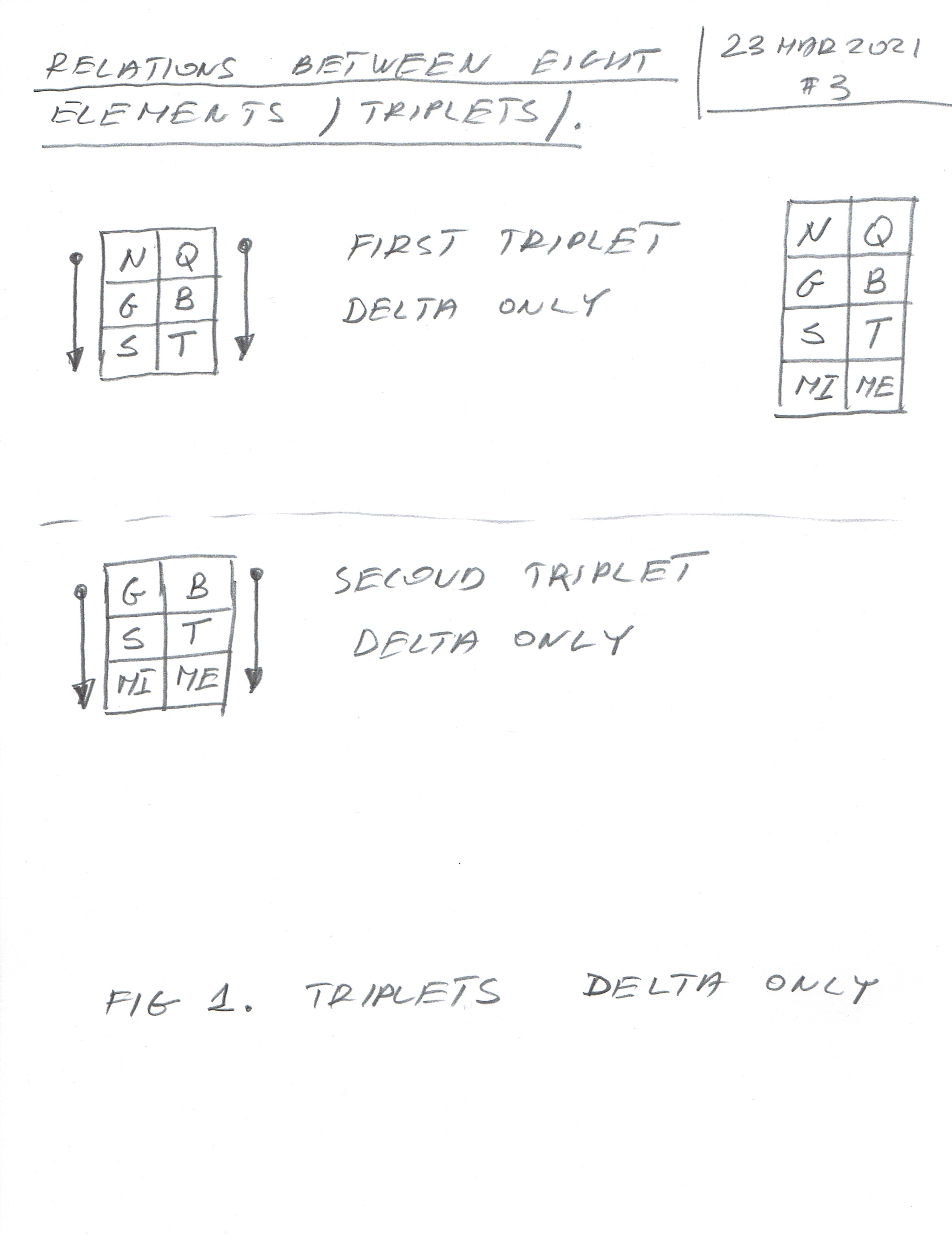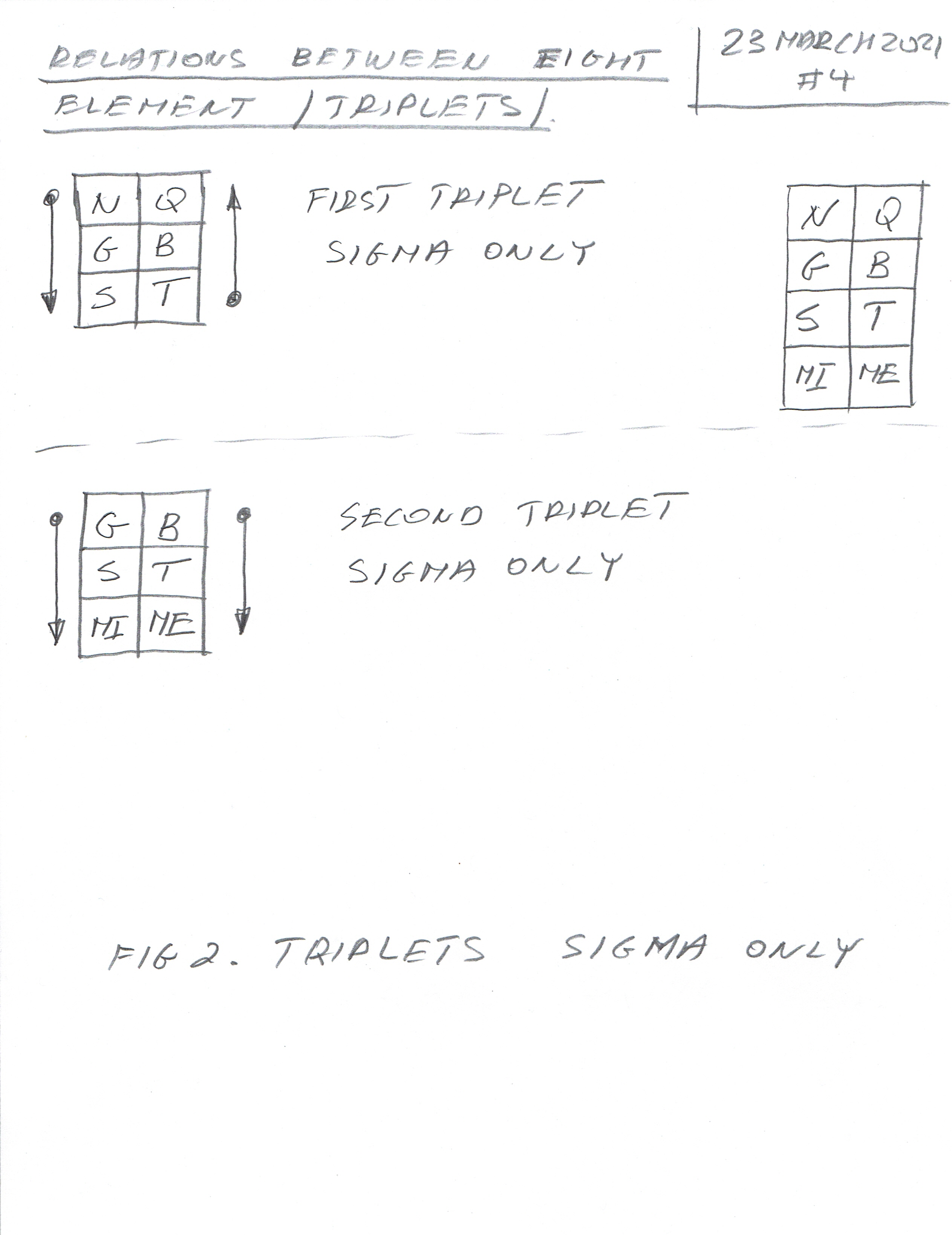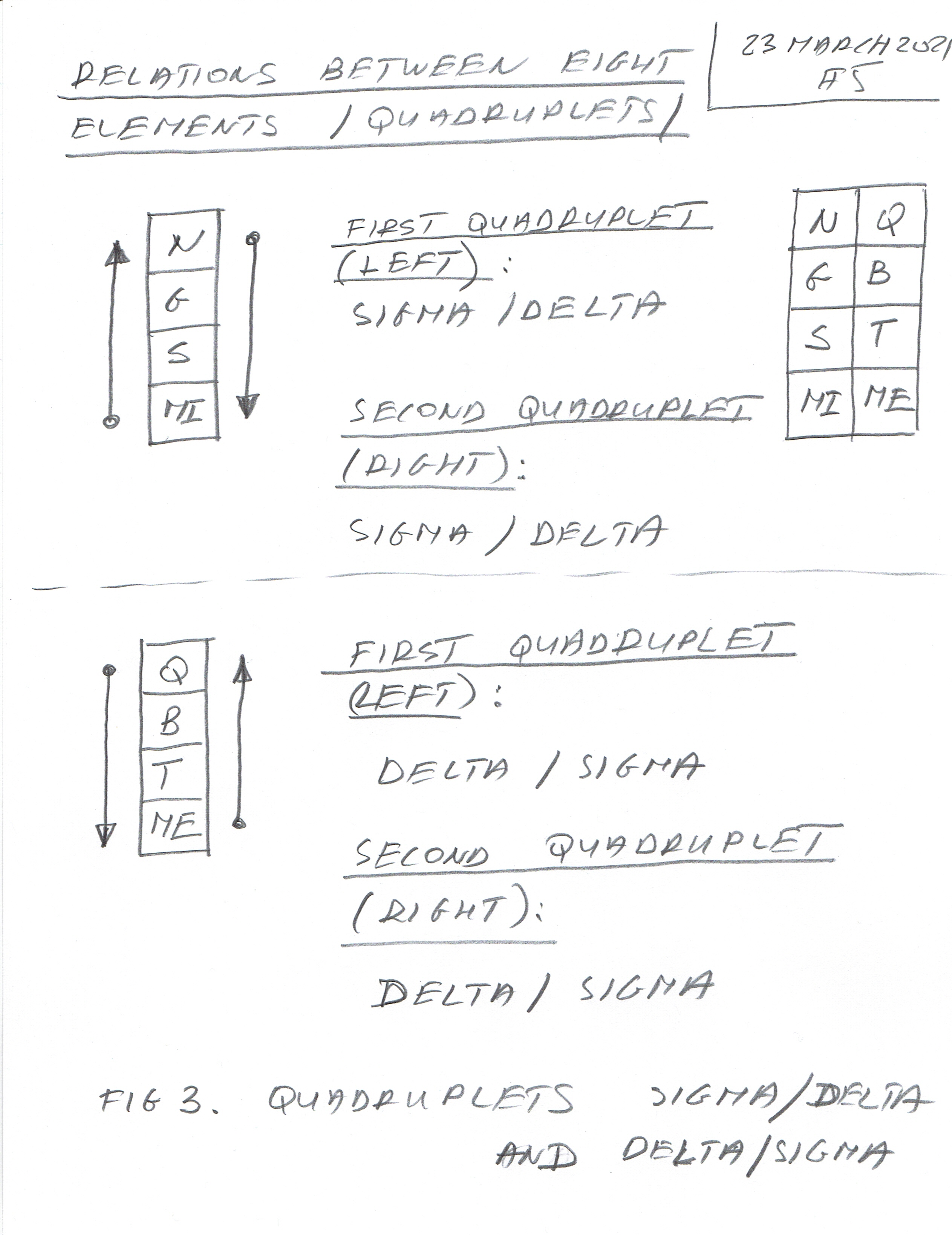This article shows symbolic relations of Triplets and Quadruplets of all Eight Elements of the Universe/Quantum - Neutrino, Graviton, Space, Membrane Internal and Quark, Boson, Time, Membrane External.

There are three self-explanatory figures showing the relation between the elements and their direction, similar to those in article 119 - Narrative for Pairs. These relations show again bonding between the Delta Angles, Sigma Angles (for triplets), and their mix (for the Quadruplets). The arrows show the direction of the interaction between the Delta CP Violating Phase Angles and Sigma Angles /Sums of three Theta Mixing (Oscillation) Angles from before and after transform/. The Sigma Transform is just the folding of the Negative Real part of the complex plane onto the positive part of the Real Plane.

In this case, we have a block of six elements (two triplets) and one complete block of eight elements forming two quadruplets.

In the following three sketches you'll see those relations and directions of interactions between the Angles.

The next articles will deal with calculating concrete values of Delta CP Violating Angles and Sigmas (sums of the Theta Mixing(Oscillation) Angles of the Eight Elements).

Figure 1 - Triplets - Delta Angles only.Figure 2 - Triplets Sigma Angles only.Figure 3 - Quadruplets - Sigma/Delat Angles and Delata/Sigma Combinations.